Mechanics

# Centripetal acceleration

Centripetal acceleration is the acceleration that causes a change in the direction of the velocity of some object that performs a circular motion . It points in the direction of the radius of motion and is calculated by the speed of the mobile squared, divided by the radius of the circle.

This acceleration arises thanks to the action of a central force , that is, a force that points to the center of a trajectory. These central forces are called centripetal forces . Since the angle between velocity and centripetal force is 90º, this force is not capable of doing work, so centripetal acceleration only changes the direction of motion, keeping the speed at which the body moves constant.

To better understand the meaning of centripetal acceleration , let’s analyze one of its possible formulas: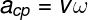cp – centripetal acceleration (m/s²)

v – speed (m/s)

According to the formula shown above, the unit of centripetal acceleration is rad/s multiplied by m/s. However, the radian unit is dimensionless (it has no unit of measurement) , so we say that centripetal acceleration is measured in m/s².

Centripetal Acceleration Formula

The most used formula for calculating centripetal acceleration is the one that relates the square of the velocity to the radius of the trajectory.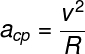v – speed (m/s)

R – radius of the trajectory

In addition to the formula above, there are variations that relate to angular quantities of circular motion, such as angular velocity or angular acceleration .

## centrifugal acceleration

When we put a wet garment to dry in a washing machine, water is expelled from the garments in the direction tangential to the displacement. About this movement, it is common to hear that centripetal acceleration is responsible for making water droplets leave the clothes, however, this statement is wrong, since inertia is responsible for the escape of water droplets .

The use of terms such as centrifugal acceleration and centrifugal force is quite common and gives the wrong idea that there is some force causing rotating bodies to tend to be thrown out of their path. This “force” that seems to push the bodies to directions that escape the center of rotation, in fact, appears in accelerating references and, therefore, is called a virtual force.

During rotation, bodies tend to continue moving in a straight line at constant speed . It is because of inertia that we can rotate a bucket full of water without its contents falling: since the centripetal force points towards the center of the trajectory, the inertia of the water makes it tend to oppose the action of this force. When the water in the bucket is close to falling, the bucket (which has less inertia) has already descended, so the water always remains in the bucket.

## Centripetal Acceleration of the Electron

A common application of centripetal acceleration is where charged particles are thrown towards a region of magnetic field . When this happens, a magnetic force acts on them, causing their trajectory to curve. In this case, the magnetic force plays the role of a centripetal force , as it constantly points towards the center of a circular path . Thus, it is sufficient to assume that the magnetic force has a centripetal character: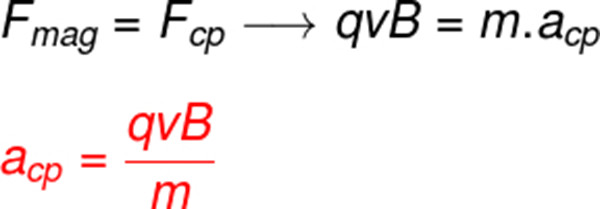mag – magnetic force (N – Newton)

cp – centripetal force (N)

q – electric charge (C – Coulomb)

v – speed (m/s)

B – magnetic field (T)

If we want to calculate the centripetal acceleration suffered by an electron, we must assume that the magnitude of q is equal to that of the fundamental electric charge (1.6 x 10 -19 C). After some adjustments, we can write a formula to calculate the radius of rotation of an electron in the presence of magnetic fields, namely: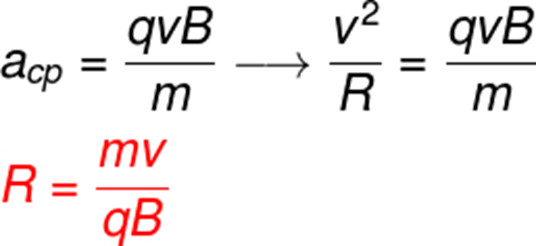Let’s make an example of applying the formula above to determine what should be the radius of the trajectory of an electron of charge 1.6 x 10 -19 C that enters a region of magnetic field of 0.5 T with a speed of 1.5 x 10 3 m/ s. The mass of electrons is approximately 9.10 -31 kg. See the calculation: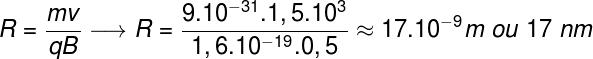You may wonder what the centripetal acceleration is of an electron orbiting the nucleus of an atom. Although pertinent, the result of this calculation leads us to physical inconsistencies and this is due to the atomic model used. If these electrons were indeed spinning, they would be constantly emitting energy, so their orbits would be unstable. Nowadays we know that electrons do not orbit atomic nuclei , but that they are distributed in the volume of the electrosphere according to much more complex laws, based on the principles of quantum mechanics

## Centripetal acceleration exercises

Question 1 – (Enem 2019) Brazil can become the first country in the Americas to join the select group of nations that have bullet trains. The Ministry of Transport foresees the launch of the international bidding notice for the construction of the Rio-São Paulo high-speed railroad. The trip will connect the 403 kilometers between Central do Brasil, in Rio, and Estação da Luz, in downtown São Paulo, in 1 hour and 25 minutes.

Due to the high speed, one of the problems to be faced when choosing the route that will be traveled by the train is the dimensioning of the curves. Assuming that a comfortable lateral acceleration for the passengers and a safe one for the train is 0.1 g, where g is the acceleration due to gravity (considered equal to 10 m/s 2 ) and that the speed of the train remains constant throughout the route, it would be correct to predict that the existing curves on the route should have a minimum radius of curvature of, approximately:

a) 80 m

b) 430 m

c) 800 m

d) 1600 m

e) 6400 m

Template: Letter E

Resolution:

We are asked to find the minimum radius of the curve that the train needs to travel so that passengers do not feel uncomfortable. For this, we must calculate the speed of the train: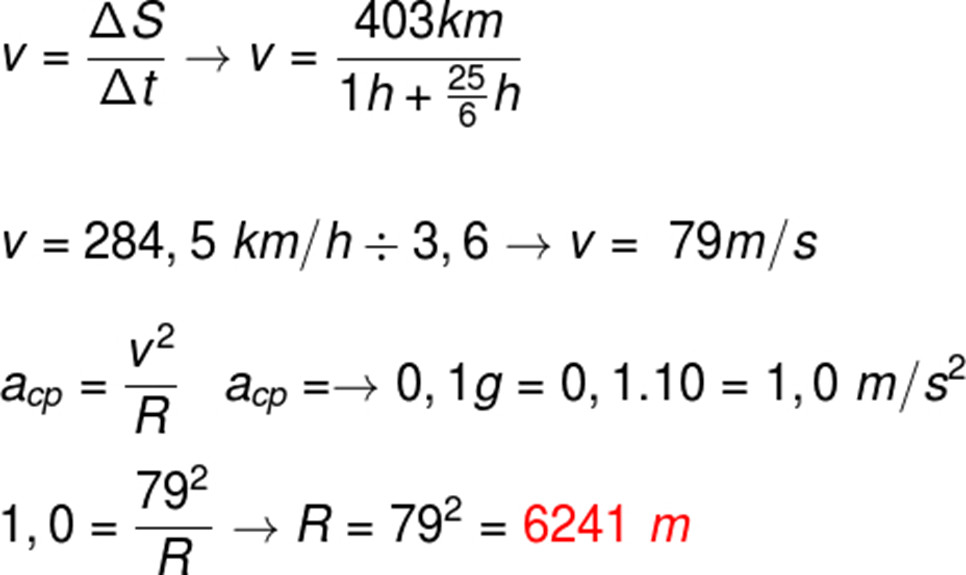Question 2 – A formula 1 car enters a curve at 108 km/h (30 m/s). Knowing that the radius of the turn in question is 90.0 m, the centripetal acceleration felt by the pilot is equal to:

a) 5 m/s²

b) 10 m/s²

c) 3 m/s²

d) 8 m/s²

e) 3 m/s²

Template: letter B

Resolution:

To do the calculation, we use the formula for centripetal acceleration. Check out: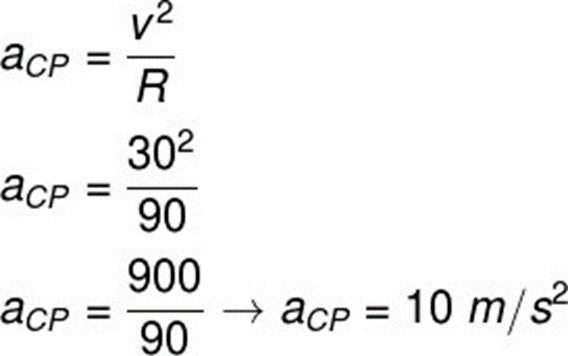According to the calculations performed, the centripetal acceleration perceived by the pilot is 10 m/s², equivalent to 1g (10 m/s², the magnitude of the acceleration due to gravity).